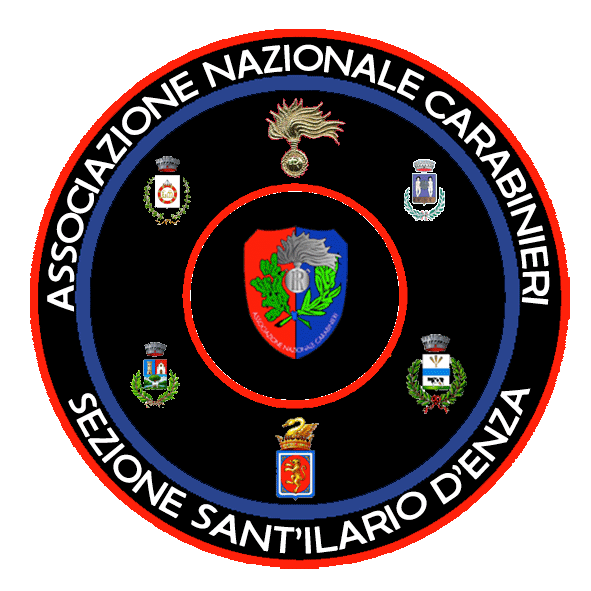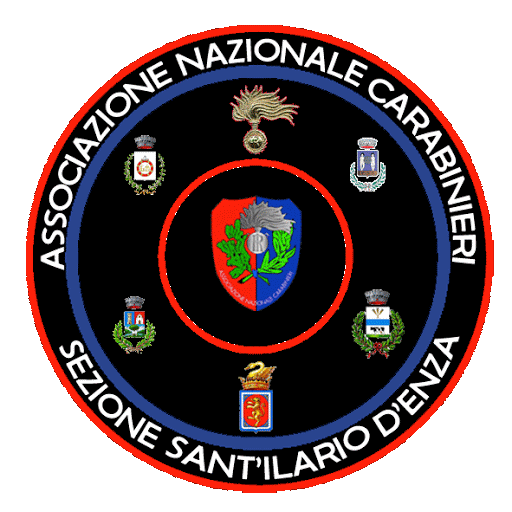ASSOCIAZIONE NAZIONALE CARABINIERI SEZIONE DI SANT'ILARIO D'ENZA (RE) 42049 - VIA ROMA NR. 22 = TEL. 349 / 6343303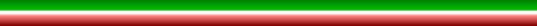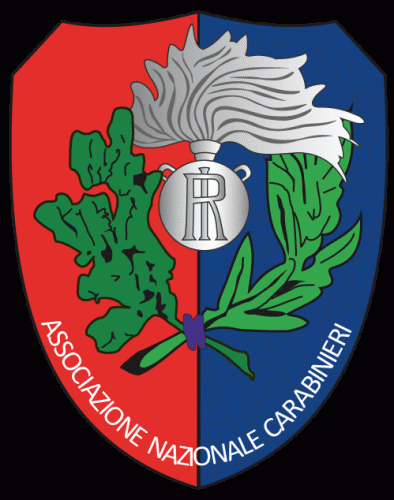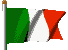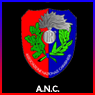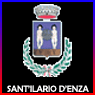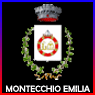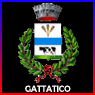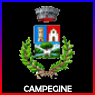=^=^=^=^=^=^=^=^=^=^=^=^=^=^=^=^=^=^=^=^=^=^=^=^=^=^=^=^=^=^=^=^=^=^= ALTRE INIZIATIVE: =^=^=^=^=^=^=^=^=^=^=^=^=^=^=^=^=^=^=^=^=^=^=^=^=^=^=^=^=^=^=^=^=^=^= =^=^=^=^=^=^=^=^=^=^=^=^=^=^=^=^=^=^=^=^=^=^=^=^=^=^=^=^=^=^=^=^=^=^=